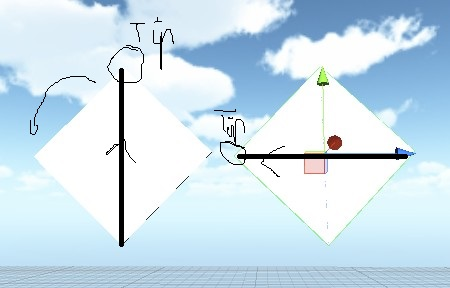# Make a cube rotate in right or left direction randomly

i have a cube which i want to rote right or left on the x axis as shown in the diagram randomly.i have seen the euler angles and the rotation concept and some questions about them but have not found them suitable for my case.my code goes like this

``````var x:float;
function Start()
{
x=Random.value;
}

function Update()
{
if(x>=0.5)
{
//rotate right
}
if(x<0.5)
{
//rotate left

}
}
``````

so i need the cube to rotate slowly towards right if the value is greater than 0.5 like in the pic attached and similarly for lesser values.Thankyou.Hey there, sorry you’re having issues with rotation.

From what I understand, you just want to randomly rotate right or left.

Here’s how to achieve that:
(not tested)

`````` var rotateRight : bool;

function Start()
{
var randomNumber = Random.Range(0,3); //Generates number between 1 & 2
if(randomNumber > 1)
rotateRight = true;
else
rotateRight = false;
}

function Update()
{
if(rotateRight)
transform.Rotate(Vector3.right * Time.deltaTime);
else
transform.Rotate(Vector3.left * Time.deltaTime);
}
``````

Use Rotate with a random range like so:

``````public float speed = 10f; //set your speed
Vector3 randomXDirection = new Vector3(Random.Range(-359, 359),0,0);

void Update (){
transform.Rotate(randomXDirection, speed * Time.deltaTime);
}
``````

Hi,

that works i tested it.

``````#pragma strict

var x:float;
var speed:float = 2.0f;

function Start()
{
x=Random.value;
}

function Update()
{
if(x>=0.5)
{
//rotate right
transform.Rotate(-speed * Time.deltaTime, 0, 0);
}

if(x<0.5)
{
//rotate left
transform.Rotate(speed * Time.deltaTime, 0, 0);
}
}
``````